´

Introduction

A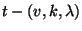-design is a set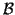of blocks of order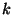on a set of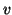vertices such that each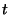-subset of the set of vertices is contained in exactly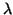blocks. More formally, let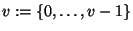denote the set of vertices and indicate by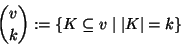the set of-subsets of the setof vertices. Thenhas to fulfill the following conditions in order to be a-design: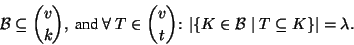The introduction of a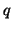-analog is now obvious: A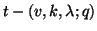-design is a setof subspaces of dimensionof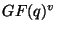such that each subspace of dimensionis contained in exactlyblocks. More formally, let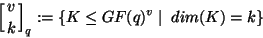indicate the set of-subspaces of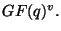Thenhas to fulfill the following conditions in order to be a-design: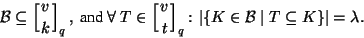As-designs are suitable selections of blocks, they can be described with the aid of the incidence matrix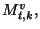the rows of which correspond to theand the columns of which correspond to the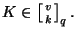The entries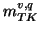of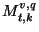are defined as follows: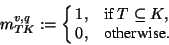Hence a-design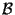is nothing but a selection of columns of that particular matrix, or, equivalently, a 0-1-vector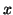which solves the system of linear equations with this particular matrix as matrix of coefficients:

1.1 Corollary   The set of-designs onis the set of selections of-subspaces that can be obtained from the 0-1-solutionsof the sytem of linear equations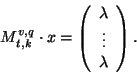The setof blocks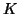of the design corresponding to the solutionis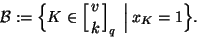There are, of course, several trivial cases, where solutions exist, for example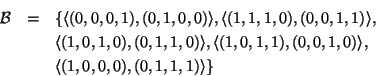is a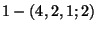design, but we are looking for nontrivial designs. The first examples were presented by S. Thomas () who found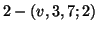-designs, for all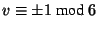. H. Suzuki () extended this family to families of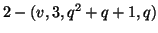-designs for arbitrary prime powersunder the same restriction onas above. As far as we know, no nontrivial-designs have been found yet for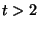.

Thomas and Suzuki used geometric arguments for their constructions, but we are interested here in a general approach that allows a systematic and complete construction of such designs (for small parameters), and therefore it has to be implemented on computers. The above lemma opens such an approach but we note that the matrix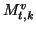is a very big matrix, so that there is not much hope to find such solutions via solving this big system of diophantine equations.

University of Bayreuth -